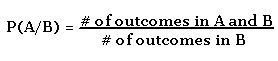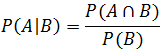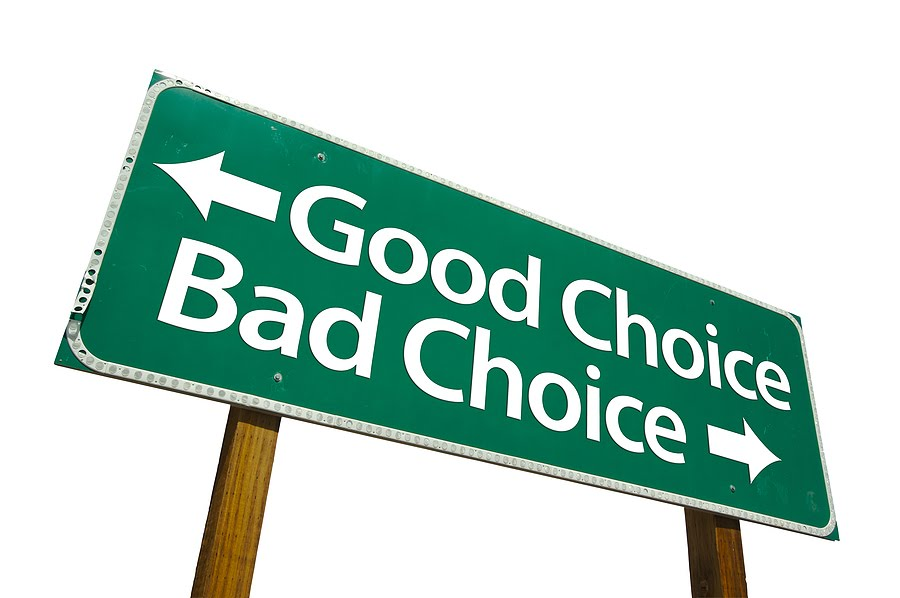Home » Solving Accuracy vs. Cost using probabilities (with case study)

# Solving Accuracy vs. Cost using probabilities (with case study)As a manager, you face cost vs. quality / accuracy trade-offs on a regular basis. This can be in the form of any of these questions:

• Should we invest more time and resources to gather incremental data and take more informed decision?
• What is the value gained by asking the analyst in your team to spend one more day on data cleansing?
• What is the incremental gain by trying out some more hypothesis and gain slightly higher lift curve in your predictive models?
• What is the value of extra time / resources spent on gathering additional information?

If you are a decision making authority, these dilemma arise quite often. Sadly, not many people have a framework to answer these questions. They end up taking these decision based on their gut or change their stand multiple times in the process.

If you do not put a framework around these problems, your decision making is dependent on how the problem is narrated to you or how you think about it at that instance. This also leads to change in stand / decisions multiple times. In this article, I have laid out a simple framework to answer these questions and have illustrated its usage through a case study.

Framework:

The framework for these situations is fairly simple. As a stand alone decision, you invest resources until the value created by these investments is higher than the cost incurred.

On the other hand, If there are multiple opportunities with limited resources, you invest in the projects, which give you the highest ROI.

Case study – Background :

You are the general manager of FUORD, an automobile company in India. FUORD has recently launched a model in India and China called Bistra. The engine of this model has been outsourced to two companies, namely X and Y (referred as vendor after this). Both X and Y make an identical design of the engines. However, 10 out of every 100 engines from Vendor X are faulty in working whereas 1 out of 100 engines of Y is faulty in working. India and China import exact the same number of engines. FUORD has a policy of not revealing the vendor name while sending the engines to any country. Hence, in any month India has an equal probability of receiving either engines manufactured by X or by Y.

Faulty engine found ::

After 10 months of launch of Bistra, you found one of the engines is of wrong design. A fault in design is against the code of conduct of FUORD and the contract with this supplier needs to be terminated immediately. But the fix is that neither you nor anyone in the firm is sure whether this engine was supplied by X or Y. Here are various costs involved in the process:

• Test for working of each engine costs the company \$40 k   .
• Cost of a decision of terminating a wrong vendor contract is \$1 MM.

Should you test an engine for its working, if it is faulty or not before terminating the supply from a vendor?

Note: Fault in design and fault in working of the engine are two independent events.

The play of probabilities:

What is the probability that the lot in India in the 10th month of the launch came from X? Obviously 0.5, as there are only two options of choosing a vendors and both equally likely. Can you take a decision to terminate any of the two vendors based on your intuition? Probably not. What do you do in such situations? Collect more information.

But is the collection of information worth the cost which you will incur to test an engine. Let’s try to find out the expected costs involved.

Event X : The lot in the 10th month is from X

Event Y : The lot in the 10th month is from Y

Event F: The chosen engine is faulty

Event P: The chosen engine is perfect

P(X) = P(Y) = 0.5

P(F/X ) = Probability of the engine being faulty given that the lot is from X = 0.1 (Given)

P(F/Y ) = Probability of the engine being faulty given that the lot is from Y = 0.01 (Given)P(F) = Probability of the engine being faulty

= P(F∩X) + P(F∩Y)

= P(F/X)*F(X) + P(F/Y)*P(Y)

= 0.5*(0.1 + 0.01) = 0.055

P(X/F) = Probability of lot being from X given that the first random engine chosen is faulty

= P(X∩F) /P(F)

= 0.5*0.1/0.055 = 0.909

P(P) = Probability of the engine being perfect  = P(P∩X) + P(P∩Y)

= P(P/X)*P(X) + P(P/Y)*P(Y)

= 0.5*(0.9 + 0.99) = 0.945

P(X/P) = Probability of lot being from X given that the first random engine chosen is perfect

= P(X∩P) /P(P)

= 0.5*0.9/0.945 = 0.47

P(Y/P) = 0.53

K : Event of choosing the correct vendor after the first engine assessment.P(K) = P(K∩F) + P(K∩P)

= P(F) * P(K/F) + P(P)*P(K/P)

= 0.055*0.909 + 0.945*0.53

= 0.05 +  0.5 = 0.55

Now let’s make some cost estimations.

Expected value of cost if engine assessment is not done= A = \$1MM * 0.5 = \$500 k

Expected value of cost if the engine assessment is done = B = \$40 k + 0.45 *\$1MM = \$490k

Clearly A > B and, hence, assessment of first engine is totally justified. Also, on a stand alone basis, we were able to reduce cost of wrong assessment by \$10 k, by investing \$40 k

End Notes :

In this part of the case study, we took a very simple case with a single step of processing. Say, you completed the first test and found the engine to be perfect. Now you wish to check if the second test is cost effective or not. Make cases and find the expected cost of test and cost saved by the test. Make the comparison and write in the box below your recommendation to do the second test or not?

You can also read this article on our Mobile APP•sumalatha says: